2021-07-13 10:36

# python,如何将生成的excel文件保存到自己选择的文件夹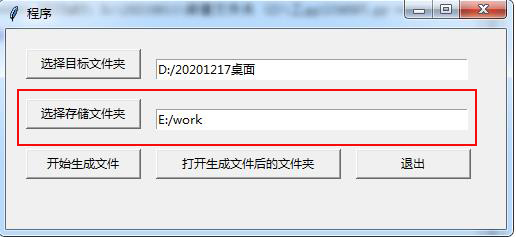• 写回答
• 关注问题
• 收藏
• 邀请回答

#### 4条回答默认 最新

•鸡蛋酱\$ 2021-07-13 11:36
已采纳

你写代码的时候，把自己要存放的路径加在save()里面就可以了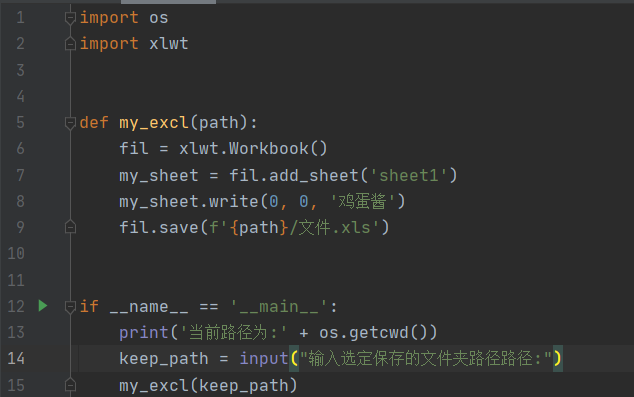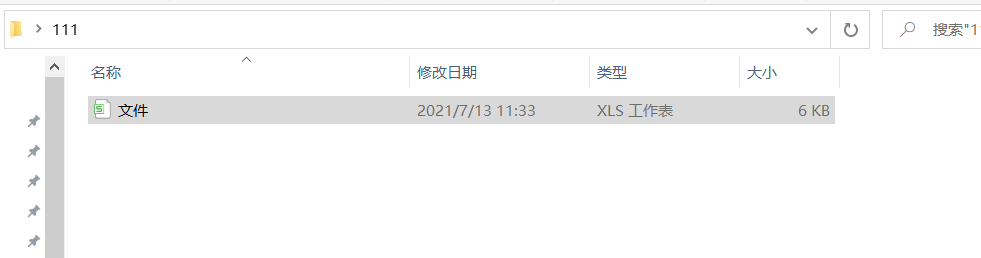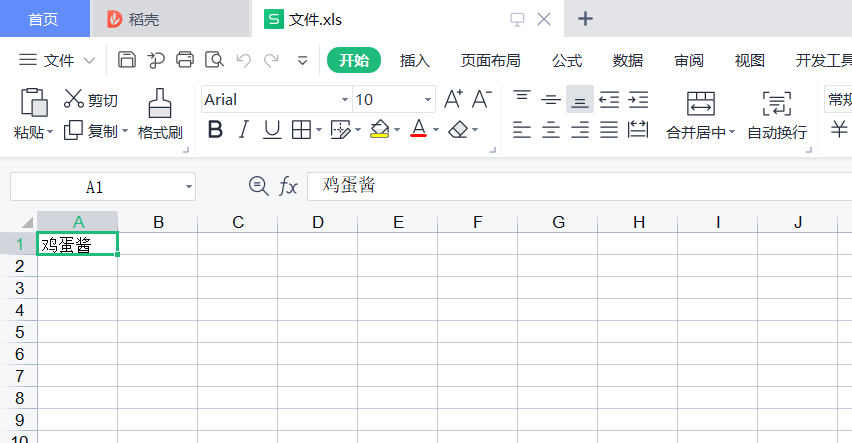已采纳该答案
1 打赏 评论
•guozhiquan89 2021-07-30 17:58

就是把你选择文件的按钮，关联一个选择路径的函数，选择路径的函数返回的路径的值赋予 文本框就好了。

``````# 按钮关联选择目录的函数
self.opendirB.clicked.connect(self.selectDirPath)
#选择目录函数
def selectDirPath(self,setPath):
path_ = QFileDialog.getExistingDirectory()
self.dirpathL.setText(path_) #目录路径赋值 文本框
``````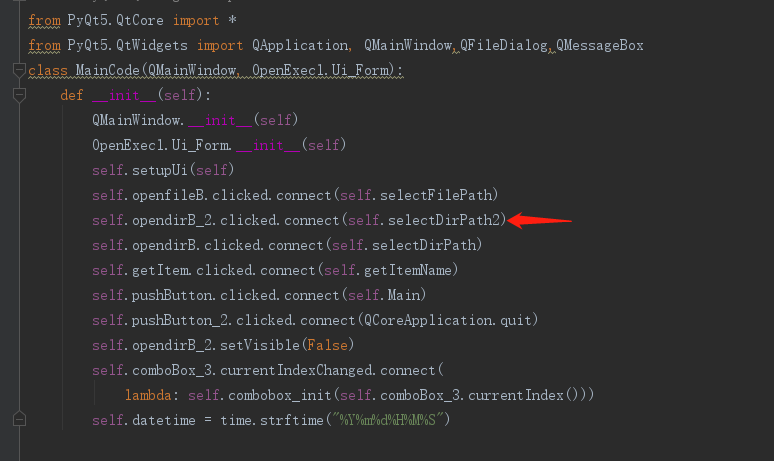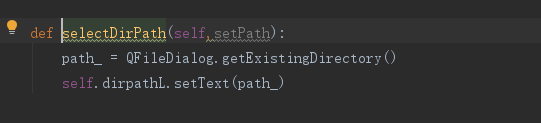1 打赏 评论
•盐湖城 2021-07-13 11:08

你选择了不就可以了吗？

打赏 评论
•weixin_55492838 2021-07-14 13:48

from tkinter import *
import tkinter as tk
from tkinter import filedialog
import os
import xlwt
import sys

class Window(Frame):

#构造函数
def init(self,master=None):

``````   Frame.__init__(self,master)
self.master=master
self.folderPath1 = tk.StringVar()
self.folderPath2 = tk.StringVar()
self.__init__window()
``````

#tkinter模块添加按钮、标签

``````def __init__window(self):
``````

#设置窗口标题

``````   self.master.title("程序")
``````

#调用pack，根据文本自适应窗口大小

``````   self.pack(fill=BOTH,expand=1)
``````

#创建按钮

``````   button1=Button(self,text='退出',width=15,height=1,command=self.client_exit)
button1.place(x=350,y=120)

button2=Button(self,text='选择目标文件夹',width=15,height=1,command=self._getFile1)
button2.place(x=20,y=20)

button3=Button(self,text='选择存储文件夹',width=15,height=1,command=self._getFile2)
button3.place(x=20,y=70)

button4=Button(self,text='开始生成文件',width=15,height=1,command=self.shengcheng)
button4.place(x=20,y=120)

button5=Button(self,text='打开生成文件后的文件夹',width=25,height=1,command=self.open_folderpath)
button5.place(x=150,y=120)
``````

#创建标签

``````   self.folderPath_en1 = tk.Entry(self,  width=44)
self.folderPath_en1.place(x=150,y=30)
self.folderPath_en1.delete(0, "end")
self.folderPath_en1.insert(0, "请选择文件夹")

self.folderPath_en2 = tk.Entry(self,  width=44)
self.folderPath_en2.place(x=150,y=80)
self.folderPath_en2.delete(0, "end")
self.folderPath_en2.insert(0, "请选择文件夹")

def _getFile1(self):
default_dir = r"文件路径"
print(self.folderPath1)
self.folderPath_en1.delete(0, "end")
self.folderPath_en1.insert(0, self.folderPath1)

def _getFile2(self):
default_dir = r"文件路径"
print(self.folderPath2)
self.folderPath_en2.delete(0, "end")
self.folderPath_en2.insert(0, self.folderPath2)
``````

#定义退出函数

``````def client_exit(self):
exit()
``````

#定义个获取目录的函数
def shengcheng(self,path):

# 创建 xls 文件对象

``````    wb = xlwt.Workbook()
``````

# 新增一个表单

``````    sh = wb.add_sheet('子目录文件信息')
``````

# 按位置添加数据，col表示列的意思

``````    dir_col=0
file_col=1
row_init=0
``````

#从配置获取目录名
dir=self.folderPath1
cclz1=self.folderPath2

``````    for parent1, dir_names1, file_names1 in os.walk(dir):
for dir_name1 in dir_names1:
for parent2,dir_names2,file_names2 in os.walk(dir+'\\'+dir_name1):
for file_name2 in file_names2:
sh.write(row_init,dir_col,dir_name1)
sh.write(row_init,file_col,file_name2)
row_init=row_init+1

wb.save(f'{path}/dir+文件夹下目录文件统计.xls')

if __name__ == '__main__':
keep_path = input(self.folderPath2)
shengcheng(keep_path)

``````

#定义打开文件目录函数

``````def open_folderpath(self):

os.startfile(str(self.folderPath2))
``````

#构造主窗体

root=Tk()

#设置主窗体大小

root.geometry("500x200")

#把root这个主窗体作为一个对象传入参数到定义的window类

app=Window(root)

#锁定窗体

root.mainloop()

打赏 评论